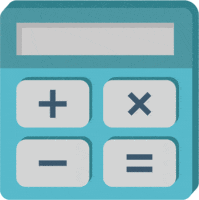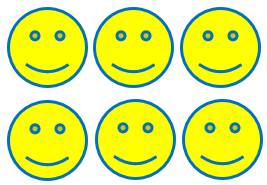# Math Worksheets Land

Math Worksheets For All Ages

# Math Worksheets Land

Math Worksheets For All Ages

Home > Math Topics > Multiplication >

# Visual Multiplication Worksheets

The beauty of math is that many of the skills overlap. When it comes to the operations addition and multiplication are our first extension of this spiral strategy to help us solve more complex problems. You can use this strategy of aligning visual objects and shapes to help students make this transition pretty quickly and easy. The basic premise is that we have students line these objects into rows and columns and then we can see the multiplication set out, but if needed you can always just count up all the objects to double check yourself. A complete series of worksheets and lessons that help students learn to multiply by using visual objects.

### Aligned Standard: 3.OA.A.1

• Answer Keys - These are for all the unlocked materials above.

### Homework Sheets

The problems here all have basic legend guides to help students along .

• Homework 1 - We count every row to get the answer.
• Homework 2 - Solve the multiplication problem using the circles as guides.
• Homework 3 - We can see that there are four rows having three images each.

### Practice Worksheets

We remove those legends and start to ask students to be more concrete with their work.

• Practice 1 - You can count rows and columns to help you through these.
• Practice 2 - Write the multiplication equation for below images and solve the problem.
• Practice 3 - Kittens and wallets in rows and columns.

### Math Skill Quizzes

The first quiz is a bit more cumulative. The second quiz focuses on a singular aspect of the skill.

• Quiz 1 - Using the letter "A", draw an equal math sentence to 5 x 3.
• Quiz 2 - Get it all going bud.Images and pictures play an important role when it comes to helping you visualize a process. They help make the problem look easier and provides a helping hand for students to get a grip of the problem. Most of the times, it is difficult for kids to transition from simple addition and subtraction to complex concepts such as multiplication and division. When they are truly just an extension of those exact operations. When learning multiplication, kids can be easily drift and start learning bad habits and the wrong concepts. This can create a problem as well as discourage and intimidate students, ultimately damaging learning outcomes.

This is where images help the students. Let's find out how images can help you learn to multiply. Colorful images are way better than abstract numbers. You don't need to spend a lot of energy and efforts in understanding the numbers. Children willingly take part in solving the problems. Images make counting and calculations of the numbers easy. Kids can easily use images as groups and multiply numbers. The images help in memorization of tables.We orientate the images into rows and columns. If we remember the form of a multiplication problem as: multiplicand x multiplier = product, this will help us further explain how to use this method. For example, if we were trying to understand how to multiply 2 and 3, we could use happy faces to do this. We would take our multiplicand (2) and create that many rows of happy faces. We will then take the multiplier (3) and create that number of columns of happy faces. As we see the product is self-evident as 6 simply by counting all the happy faces. This simple method really helps students that are having trouble transitioning from addition to multiplication operations.

Unlock all the answers, worksheets, homework, tests and more!
Save Tons of Time! Make My Life Easier Now

## Thanks and Don't Forget To Tell Your Friends!

I would appreciate everyone letting me know if you find any errors. I'm getting a little older these days and my eyes are going. Please contact me, to let me know. I'll fix it ASAP.